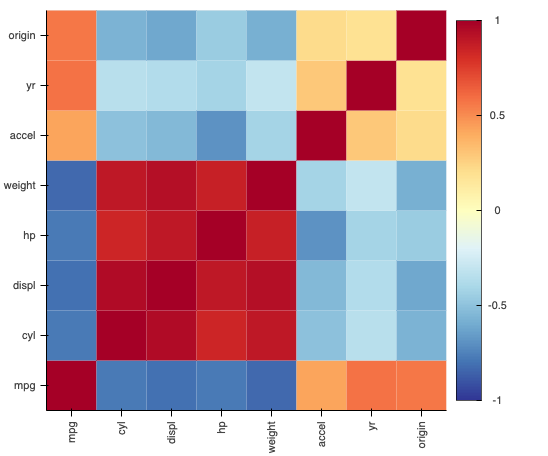# Diagonal HeatMap for Pearson or Covariance values

Is it possible to make an heatmap with correlation values of the data, like this one in the picture below?

The easiest way to generate a correlation matrix is to use pandas to compute it and then construct it like this:

``````import pandas as pd
from bokeh.sampledata.autompg import autompg
corr = autompg.corr()
hv.HeatMap((corr.index, corr.columns, corr.values))
``````